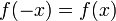# Even function

An even function is a function$f$ defined on a subset of the reals satisfying the following two conditions:
1. The domain of the function is symmetric about 0, i.e., for every$x$ in the domain,$-x$ is also in the domain.
2. For every$x$ in the domain, we have the equality:$\! f(-x) = f(x)$